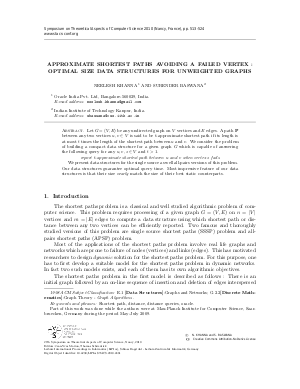Document# Approximate Shortest Paths Avoiding a Failed Vertex: Optimal Size Data Structures for Unweighted Graphs

### Authors Neelesh Khanna, Surender Baswana## File

LIPIcs.STACS.2010.2481.pdf
• Filesize: 329 kB
• 12 pages

## Cite As

Neelesh Khanna and Surender Baswana. Approximate Shortest Paths Avoiding a Failed Vertex: Optimal Size Data Structures for Unweighted Graphs. In 27th International Symposium on Theoretical Aspects of Computer Science. Leibniz International Proceedings in Informatics (LIPIcs), Volume 5, pp. 513-524, Schloss Dagstuhl - Leibniz-Zentrum für Informatik (2010)
https://doi.org/10.4230/LIPIcs.STACS.2010.2481

## Abstract

Let $G=(V,E)$ be any undirected graph on $V$ vertices and $E$ edges. A path $\textbf{P}$ between any two vertices $u,v\in V$ is said to be $t$-approximate shortest path if its length is at most $t$ times the length of the shortest path between $u$ and $v$. We consider the problem of building a compact data structure for a given graph $G$ which is capable of answering the following query for any $u,v,z\in V$ and $t>1$. \centerline{\em report $t$-approximate shortest path between $u$ and $v$ when vertex $z$ fails} We present data structures for the single source as well all-pairs versions of this problem. Our data structures guarantee optimal query time. Most impressive feature of our data structures is that their size {\em nearly} match the size of their best static counterparts.
##### Keywords
• Shortest path
• distance
• distance queries
• oracle

## Metrics

• Access Statistics
• Total Accesses (updated on a weekly basis)
0